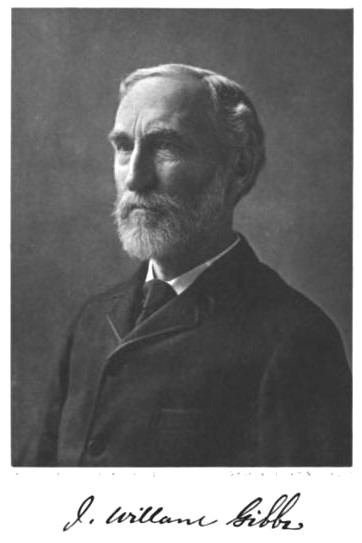Chapter 18. Chemical Thermodynamics

# Gibbs Free Energy

Jessie A. Key

### Learning Objectives

• To gain an understanding of Gibbs free energy.
• To understand the relationship between the sign of Gibbs free energy change and the spontaneity of a process.
• To be able to determine Gibbs free energy using standard free energies of formation.

Figure 18.4. J. Willard GibbsA portrait of J. Willard Gibbs

J. Willard Gibbs (1839-1903) proposed a single state function to determine spontaneity:

G = H – TS

where H is the enthalpy of the system, S is the entropy of the system, and G is Gibbs free energy.

The change in Gibbs free energy, ΔG, is the maximum amount of free energy available to do useful work. For an isothermal process, it can be expressed as:

ΔG = ΔH – TΔS                      or at standard conditions:            ΔG = ΔH⁰  TΔS

This single term, Gibbs free energy (G), allows us to avoid calculating the entropy of the surroundings. It is really just a simplification of our previous method of estimating spontaneity:

ΔSuniverse = ΔSsys + ΔSsurr$\Delta\textit{S}{}_{universe}$ =$\Delta \textit{S}{}_{sys}$ + ($\frac{{-{\rm \ }\Delta {\rm H}}_{sys}}{T}$ )

Multiply both sides of the equation by –T:

TΔSuniverse=$\Delta\textit{S}{}_{sys}$ + ($\frac{{-{\rm \ }\Delta {\rm H}}_{sys}}{T}$ )

TΔSuniverse = ΔHsysTΔSsys

Therefore ΔG = TΔSuniverse

As a result of this relationship, the sign of Gibbs free energy provides information on the spontaneity of a given reaction:

If ΔG > 0, the reaction is nonspontaneous in the direction written.

If ΔG = 0, the reaction is in a state of equilibrium.

If ΔG < 0, the reaction is spontaneous in the direction written.

The significance of the sign of a change in Gibbs free energy parallels the relationship of terms from the equilibrium chapter: the reaction quotient, Q, and the equilibrium constant, K.

If Q > K, the reaction is nonspontaneous in the direction written.

If Q = K, the reaction is in a state of equilibrium.

If Q < K, the reaction is spontaneous in the direction written.

Example 4

Calculate ΔG⁰ for a reaction where ΔH⁰ is equal to 36.2 kJ and ΔS⁰ is equal to 123 J/K at 298 K. Is this a spontaneous reaction?

Solution

ΔG = ΔH⁰ – TΔS

ΔG = 36.2 kJ (298 K x 123 J/K)

ΔG = -0.4 kJ

Therefore the reaction is spontaneous because ΔG⁰ is negative.

## Determining ΔG⁰ from Standard Free Energy of Formation

The standard Gibbs free energy change, ΔG⁰, for a reaction can be calculated from the standard free energies of formation, ΔGf .

ΔGf  = ∑nΔGf(products) – ∑mΔGf(reactants)

where n and m are the coefficients in the balanced chemical equation of the reaction.

Standard free energies of formation values are listed in the appendix, “Standard Thermodynamic Quantities for Chemical Substances at 25°C.”

Example 5

Calculate the standard free energy change for the following reaction, using standard free energies of formation:

5 C(s) + 2 SO2(g) → CS2(g) + 4 CO(g)

Is this a spontaneous reaction?

Solution

ΔG⁰= ∑nΔGf(products) – ∑mΔGf(reactants)

ΔG⁰ = [(4 x −137.2 kJ/mol) + (67.1 kJ/mol)] – [(5 x 0 kJ/mol) + (2 x −300.1 kJ/mol)]

ΔG⁰= (-481.7 kJ/mol) – (-600.2 kJ/mol)

ΔG⁰= 118.5 kJ/mol

ΔG⁰ has a positive value so this is not a spontaneous process.

### Key Takeaways

• The change in Gibbs free energy (ΔG)  is the maximum amount of free energy available to do useful work.
• If Δ> 0, the reaction is nonspontaneous in the direction written. If Δ=0, the reaction is in a state of equilibrium. If Δ< 0, the reaction is spontaneous in the direction written.
• The standard Gibbs free energy change, ΔG⁰, for a reaction can be calculated from the standard free energies of formation, ΔGf.

1. Gibbs Josiah Willard/Public Domain

## LicenseGibbs Free Energy by Jessie A. Key is licensed under a Creative Commons Attribution 4.0 International License, except where otherwise noted.